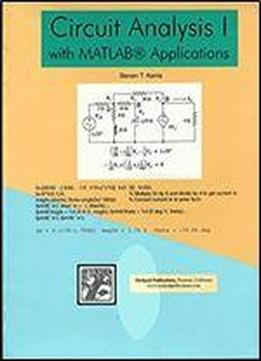# Circuit Analysis I With Matlab Applications, Illustrated Edition by Steven T. Karris / 2003 / English / PDF

This introduction to the basic principles of electrical engineering presumes basic knowledge of differential and integral calculus and physics. Students learn the fundamentals of electrical circuit analysis and are introduced to MATLAB—software used to write efficient, compact programs to solve electrical and computer engineering problems of varying complexity.

views: 369